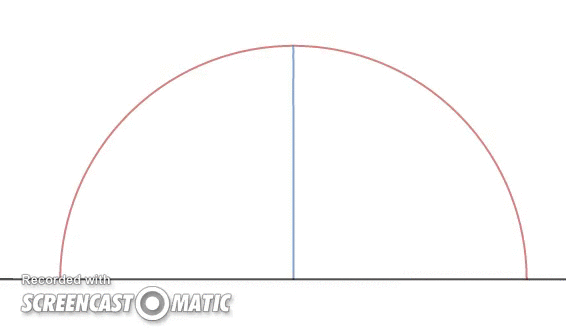# You wanted a Staircase Problem Trevor?

Geometry Level 4Trevor is at the edge and inside of a circular semicircle of radius 1. He walks a distance of $d$ towards the radius of the semicircle and climbs up until his head hits the top of the semicircle. Then he walks another distance of $d$ maintaining the same height as when he hit his head the first time and climbs up again until his head hits the semicircle. This continues like a staircase pattern and when he reaches the top, he stops and shouts in victory. (The GIF shows an illustration of it)

Let $d=\frac{1}{n}$ where $n$ is an integer more than 0 and $D_{n}$ be the total staircase-zigzag distance traveled by Trevor.

Find

$\lim _{ n\rightarrow \infty }{ D_{n} }$

Assume Trevor has a height of $0$

×